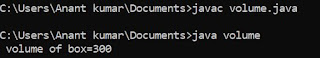## Friday, 1 June 2018

### Program to find out square of first n odd number in c

/* program to square of n odd number */
# include <stdio.h>
void main ()
{
int a=1,n,b,counter ;
clrscr () ;
printf ("\n how many number you want to square:") ;
scanf ("%d", & n) ;
for (counter=0; counter<n; counter++)
{
b = a*a ;
printf ("%d", b) ;
a =a+2 ;
}
getch () ;
}